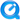Intermediate Algebra (RCC - Math 95)
- TI-83/84 Help - MyOpenMath Login - Desmos Graphing Calculator - Completed Notes- Syllabus

Jump to : Unit 10 - Unit 11 - Unit 12 - Final Review

 Unit 8 : Roots and Radicals Lesson Handouts Completed Notes Support Resources Videos available as Quicktimedownload or on8.1: Simplify Expressions with Roots Quiz #1: 8.1-8.4 Solutions 8.2: Simplifying Radical Expressions 8.3: Simplifying Rational Exponents Simplifying rational exponents examples8.4: Add, Subtract, and Multiplying Radical Expressions 8.5: Dividing Radical Expressions Quiz 8.4-8.8 Solutions Simplifying Expressions with radicals(These are the extra examples from class on 10/6) 8.6: Solving Radical Equations Solving Radical Equations Examples 8.7: Using Radical Functions 8.8: The Complex Numbers Multiplying and Dividing Complex Numbers videoUnit 8 Review
 Unit 9: Quadratic Functions and Equations Lesson Handouts Completed Notes Support Resources Videos available as Quicktimedownload or on9.1: Solving Quad. Equations using Square Root method Solving Quadratic Equations Part 1: Square Root Method9.2 Solving Quad. Equations by Completing the Square Solving Quadratic Equations: Part 2: Completing The Square9.3 Solving Quad. Equations by the Quadratic Formula Solving Quadratic Equations Part 3: The Quadratic Formula9.4 Solving Equations in Quadratic Form 9.5 Solve Applications of Quadratic Equations 9.6 Graph Quadratic Functions using Properties Desmos Exploration Axis of Symmetry from Standard Form.9.7 Graph Quadratic Functions using Transformations Changing a Function from standard form to Vertex Form (Method 1)Vertex Form Example videoUnit 9 Review Ch 9 Review Handout (with answers) Last Years Review (very similar) and Answers video;You can use this video to find practice problems that you are unsure about. Sales Plan Project Sales Plan Project handout Write or type up your sales plan and show the supporting mathematics. Desmos.com - You can use this to make a nice graph of your functions.
 Unit 10: Exponential Functiosn and Logarithmic Functions Lesson Handouts Completed Notes Support Resources Videos available as Quicktimedownload or on YouTube10.1 Composite and Inverse Functions Quiz #5 (10.1-10.3) Solutions 10.2 Exponential Functions Exponential Functions - Part 1:Exponential Functions (16:12)Graphing Exponential Functions video10.3 Logarithmic Functions Graphing Logarithmic Functions video10.5: solving Exponential and Logarithmic Equations Solving Exponential Equations (with a little log review)Unit 10 Review Review Handout (with Solutions)